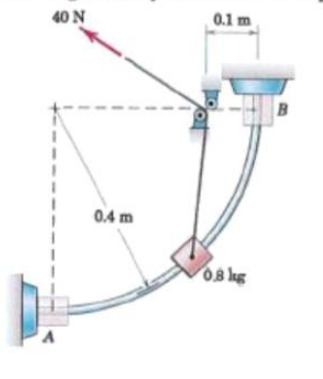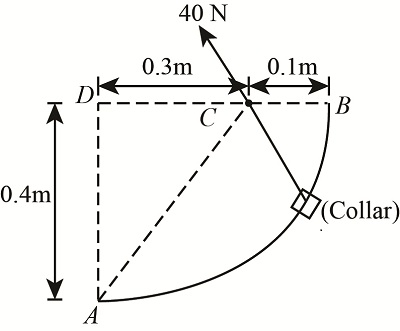# The 0.8-kg collar slides freely on the fixed circular rod. Calculate the velocity v of the collar...

## Question:

The 0.8-kg collar slides freely on the fixed circular rod. Calculate the velocity v of the collar as it hits the stop at B if it is elevated from rest at A by the action of the constant 40-N force in the cord. The cord is guided by the small fixed pulleys.## Energy

Energy represents the capacity of the body or system to do useful work. The mathematically represents the energy is multiplication force with displacement of body. Its unit is Newton-Meter.

## Answer and Explanation:

Given Data:

• The mass of collar slide is: {eq}{m_c} = 0.8\;{\rm{kg}} {/eq}
• The constant force act in cord is: {eq}F = 40\;{\rm{N}} {/eq}
• The vertical distance from point A to B is: {eq}{l_1} = 0.4\;{\rm{m}} {/eq}
• The distance between point D to C is: {eq}{l_2} = 0.3\;{\rm{m}} {/eq}
• The initial velocity of collar which is at rest is: {eq}V{ _1} = 0 {/eq}
• The final velocity of collar is: {eq}V{ _2} {/eq}

The diagram of given problem isThe expression for distance between point A to C , initial to final condition is:

{eq}{l_d} = \sqrt {l_1^2 + l{}_2^2} {/eq}

Substitute the value in above expression

{eq}\begin{align*} {l_d} &= \sqrt {{{\left( {0.4\;{\rm{m}}} \right)}^2} + {{\left( {0.3\;{\rm{m}}} \right)}^2}} \\ {l_d} &= 0.5\;{\rm{m}} \end{align*} {/eq}

The collar goes from point A to B so it gain potential energy. The expression for potential energy of collar is

{eq}{E_c} = {m_c}{g_o}{l_1} {/eq}

The collar posses initial and final velocity. So it has kinetic energy.

The expression for initial kinetic energy is

{eq}{K_{ei}} = \dfrac{1}{2}{m_c}V_1^2 {/eq}

The expression for final kinetic energy is

{eq}{K_{e2}} = \dfrac{1}{2}{m_c}V_2^2 {/eq}

The expression for change in kinetic energy from initial to final position is

Change in kinetic energy is equal to final kinetic energy minus initial kinetic energy.

{eq}\begin{align*} \Delta {K_e} &= {K_{e2}} - {K_{e1}}\\ \Delta {K_e} &= \dfrac{1}{2}{m_c}V_2^2 - \dfrac{1}{2}{m_c}V_1^2 \end{align*} {/eq}

The force in cord produces so due to this force and with its length it also posses energy.

The expression for energy generate by cord force is

{eq}{E_d} = F{l_d} {/eq}

The expression for energy balance is

{eq}\begin{align*} F{l_d} - {E_c} &= \Delta {K_e}\\ F{l_d} - {m_c}{g_o}{l_1} &= \dfrac{1}{2}{m_c}V_2^2 - \dfrac{1}{2}{m_c}V_1^2 \end{align*} {/eq}

Substitute the value in above expression

{eq}\begin{align*} 40\;{\rm{N}} \times 0.5\;{\rm{m}} - 0.8\;{\rm{kg}} \times 9.81\;{\rm{m/}}{{\rm{s}}^{\rm{2}}} \times 0.4\;{\rm{m}} &= \dfrac{1}{2} \times 0.8\;{\rm{kg}} \times V_2^2 - \dfrac{1}{2} \times 0.8\;{\rm{kg}} \times {\left( 0 \right)^2}\\ {V_2} &= 5.511\;{\rm{m/s}} \end{align*} {/eq}

Thus the velocity of collar when it reaches the point B is {eq}5.511\;{\rm{m/s}} {/eq}

#### Learn more about this topic:What is Energy Conservation? - Definition, Process & Examples

from ICSE Environmental Science: Study Guide & Syllabus

Chapter 1 / Lesson 6
92K